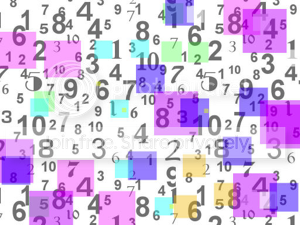0

## The Mystery of 24

Published on Thursday, May 02, 2013 in , ,The other day, I ran across an unusual mathematical fact which I had never encountered before.

Take any prime number that's equal to or greater than 5, square it (multiply it by itself), and then subtract 1. The result will always be evenly divisible by 24! Now for the hard part: Why does that always work?

Let's take a look at closer at this with our favorite computational knowledge engine. Wolfram|Alpha accepts the command prime[x], in which x is any whole number, and the prime command returns the xth prime number. For example, prime returns 2, because 2 is the 1t prime number. Prime returns 3, prime returns 5, and so on.

We'll have Wolfram|Alpha take the 3rd through the 13th prime number, square each of them, subtract 1, and then divide by 24. Sure enough, every one of those is evenly divisibly by 24!

To find out why this happens, we need to break the problem down into its basic parts.

Squaring and subtracting 1: The first step in the problem, of course, is squaring and then subtracting 1. The mathematical way to write this is x2 - 1. Not only can this be factored, but it happens to be a very standard and simple problem known as factoring the difference of 2 squares.

Working this particular problem out, we get: x2 - 1 = (x + 1)(x - 1). In plain English, squaring any number x and subtracting 1 is the same as multiplying a number 1 greater than x by a number 1 less than x. As a practical example, 62 - 1 = 36 - 1 = 35. Our factoring tells us we should be able to get the same answer by multiplying (6 + 1)(6 - 1) = (7)(5) = 35, so sure enough, that works!

Regular Grey Matters readers will recognize this principle from the Squaring 2-Digit Numbers Mentally tutorial in the Mental Gym.

Now that we've established that squaring a number and subtracting 1 is the same a multiplying the two numbers immediately above and below that number, it's time to examine the other parts of this problem.

Using odd numbers: Every prime number equal to or greater than 5 will be an odd number, of course. What happens as a result of starting with odd numbers?

When taking the numbers immediately above and below any whole odd number, you're naturally going to wind up with 2 even numbers with a difference of 2. If you start with 15, then you'll effectively be multiplying 14 by 16. Starting with 17 results in multiplying 16 by 18, and starting with 19 results in 18 by 20.

With 2 even numbers, it shouldn't be surprising that x2 - 1 will result in a number evenly divisible by 4. But if we take a closer look, there's something even more interesting to discover.

Every other multiple of 2 is also a multiple of 4. So, when you're multiplying any 2 even numbers with a difference of 2, you're always multiplying a multiple of 4 by a multiple of 2.

In other words, when starting with any odd number as x, and running it through the formula x2 - 1, you'll always wind up with a number which is evenly divisible by 8!

That may explain the 8, but 24 ÷ 8 = 3. Why does running prime numbers through x2 - 1 result in numbers divisible by 3, as well?

Using prime numbers: When considering any group of 3 consecutive whole numbers, exactly 1 of those numbers must be a multiple of 3. With 15, we're considering the numbers 14, 15, and 16, for example, and 15 is the only one of those which is divisible by 15.

However, when we limit our choice for x to prime numbers equal to or greater than 5, we're guaranteeing that our odd number itself will not be a multiple of 3. If it were, it wouldn't be a prime number by definition.

That means when we deal with the numbers immediately above and below a prime number, one of those even numbers must be a multiple of 3. Starting from 17, we're multiplying 16 by 18, and 18 is the multiple of 3. With 19, we're multiply 18 by 20, and with 23, we're multiplying 22 by 24.

Choosing prime numbers effectively forces us to multiply a multiple of 4 by a multiple of 2, and also requires that one of those even numbers be multiple of 3.

What the problem really boils down to is the request to choose any odd number x which isn't a multiple of 3, multiply (x + 1)(x - 1), and the result will always divisible by 24 for the reasons I've detailed above.

Since prime numbers equal to or greater than 5 all fit this definition, they will all work. The trick is that there are other odd numbers which will work, as well.

49, for example, isn't a prime number, yet it's still an odd number which isn't a multiple of 3. (492 - 1)= (49 + 1)(49 - 1) = (50)(48) = 2400, and even without a calculator handy, I'm pretty sure that's evenly divisible by 24.

Sometimes, it's fun just to wander through the forest of mathematics, and discover treasures like this.